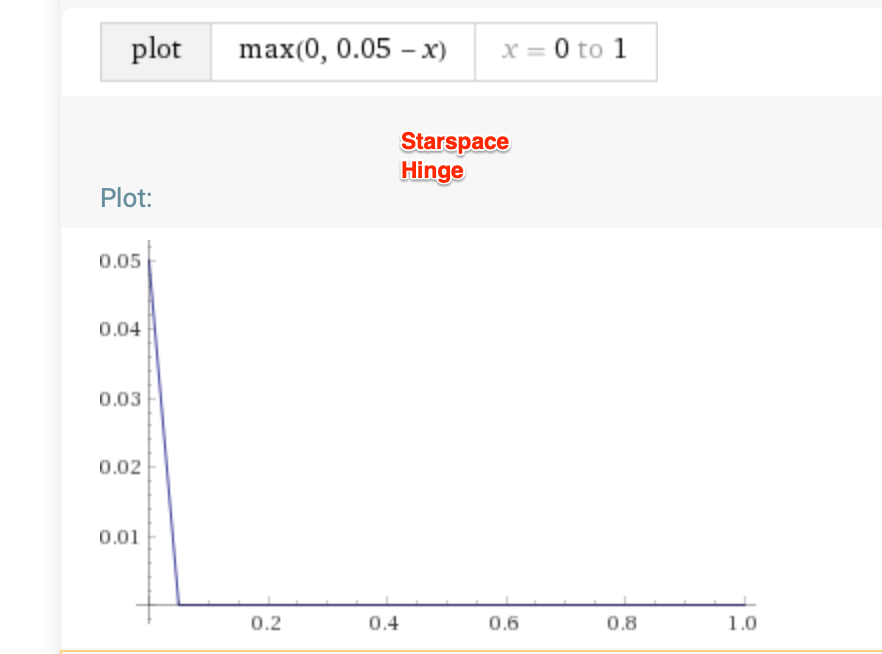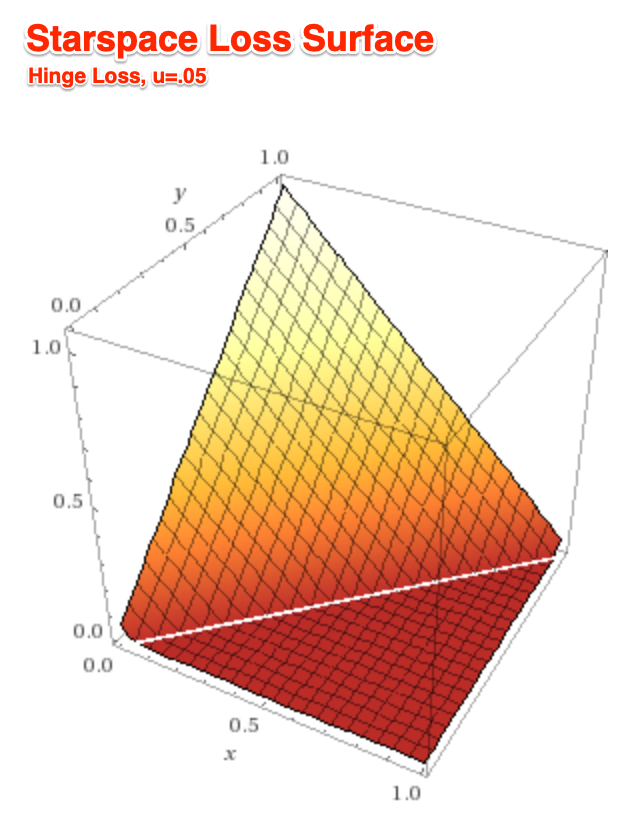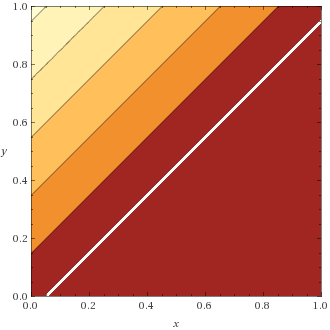Alex Egg,

# Starspace

Entities, such as document or a user are represented by a bag of features (variable sized) such as words or liked items respectively.

An entity $a$ is represented by the sum of its features: $\sum_{i\in a} F_i$ where $F$ is a feature matrix $D \text{x} d$ (interesting that it’s purely additive and not averaged)

The loss is formulated:

So they generate two sets of pairs: the positive set $(a,b)$ and the negatives $(a,b^-)$ where the number of negative pairs is controlled by the hyper parameter $k$ . For example, text classification, $a$’s could be documents and $b$’s could be labels, then we’d have a bunch of negative pairs to compare against a positive pair.

$sim(x,y)$ Is simply a distance metric eg dot, cos. In other words 1 means similar and 0 means disimiliar.

The loss then compares the positive pair w/ the negative pairs. They propose triplet hinge loss or softmax: